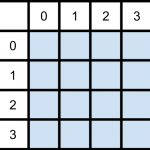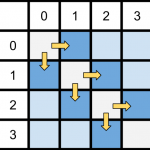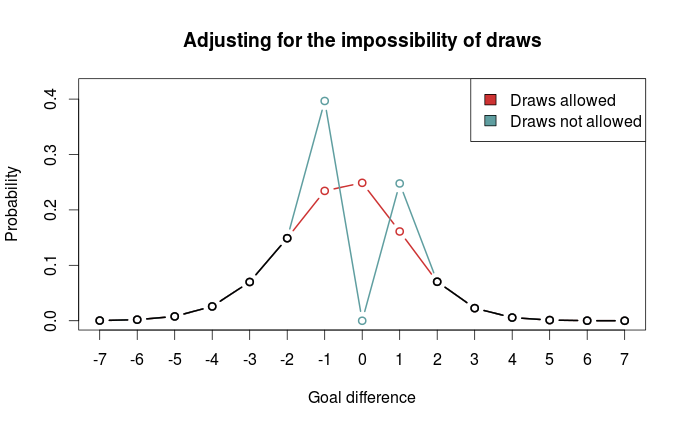# Some thoughts on goal differences in football matches without draws

In regular league matches, draws are a common occurrence. Modeling and predicting draws have some complications. Elo-type ratings allows for draws by simply treating them as half-wins for each team, but it does not allow for direct calculation of draw probabilities. Poisson regression models naturally lets you figure out the probability of a draw by calculating the probability of a goal difference of zero.

Poisson models have the additional strength over Elo-type systems in that they can be used to model and predict the number of goals scored, not only who wins (or loose, or draws). The models I have looked at all assumes that draws are possible, and that is the case in regular league matches. But what about the matches where draws are not allowed, such as in knockout tournaments? How could you calculate probabilities for different number of goals?

I haven’t really seen any discussion about this anywhere, but I have this one idea I just want to get out there. Bear in mind that this idea I present here is completely untested, so I can not say for sure if it is any good.

Matches where draws are impossible are a minority of the matches, so building and fitting a separate model for just those matches is not a good idea. Instead I propose an adjustment to be applied for just those matches. The adjustment can be motivated as follows: The game starts with 0-0 between the teams, so at least one goal has to be scored. This should increase the probabilities of 0-1 and 1-0 results. Similar argument can be given to a game that is in a 1-1 state, a 2-2 state, and so on; at least one goal has to be scored.

So the adjustment is to simply divide the probabilities for a draw and add them to the probabilities for a one-goal difference. This should of course be illustrated with an example.

Suppose you have a matrix with goal probabilities. This can be computed using a Poisson regression model, perhaps with the Dixon-Coles adjustment or some other bivariate structure, or perhaps it comes from a completely different kind of model. It doesn’t really matter.Then it is just to divide the draw probabilities and add them to the appropriate cells in the matrix:But how should the probabilities be divided? We could just divide them evenly between the two teams, but I think it is more appropriate to divide them based on the relative strengths of the two teams. There are may ways this could be done, but I think a reasonable method is to divide them based on the win-probabilities for the two teams (given that there is no draw). This does not rely on anything other than the goal probability matrix itself, and is easy to compute: Take the sum of the upper and lower triangle of the matrix, divided by the sum of the whole matrix except the diagonal. This also maintains the original win/lose probabilities.

This scheme is easy to implement in R. First we need a matrix of probabilities, which I here just compute using two Poisson distributions, then calculate the win probability of the team with goals on the vertical. After that we divide the diagonal with the win-probabilities.

```# Matrix of goal probabilities
probability_matrix <- dpois(0:7, 1.1) %*% t(dpois(0:7, 1.6))

# Win probabilities, for dividing the draw probabilities
prop <- sum(mm[lower.tri(mm)]) / (1 - sum(diag(mm)))

# Diagonal values, split proportionally
divided_vertical <- (diag(probability_matrix) * prop)
divided_horizontal <- (diag(probability_matrix) * (1-prop))
```

Here we encounter a problem. The two vectors we are going to add to the two secondary diagonals are one element too long. If we have a big enough probability matrix, that last element is probably going to be so small that ignoring it should not matter too much.

```# Increase the probabilities for one-goal wins.
diag(probability_matrix[-1,]) <- diag(probability_matrix[-1,]) + divided_vertical[-length(divided_vertical)]
diag(probability_matrix[,-1]) <- diag(probability_matrix[,-1]) + divided_horizontal[-length(divided_horizontal)]

# The main diagonal, with draw probabilities, should be 0.
diag(mm) <- 0
```

As always, it is nice to see how the probabilities of the goal differences are distributed. Here I have plotted the adjusted and unadjusted probability distributions:We clearly see that one-goal wins are much more probable.

As I mentioned above, I haven’t really looked at any data, and it is quite possible that other adjustments are better. Perhaps boosting one-goal wins is a poor idea, and spreading the probabilities more out would be better.